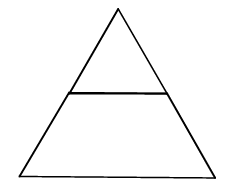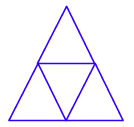### Home > ACC6 > Chapter 2 Unit 2 > Lesson CC1: 2.2.1 > Problem2-24

2-24.

Lakisha made a giant triangle using two pattern blocks: one green triangle and one red trapezoid.1. What is the area of the entire shape if the green triangle has an area of $1$ square cm?

How does the green triangle relate to the size and shape of the trapezoid? Try drawing the trapezoid with only triangles.

Draw the entire shape with only triangles.Since you know that the original triangle is $1$ square cm, and you now have $4$ triangles, then the entire area is $4$ square cm.

$4$ square cm.

2. What is the area of the entire shape if the red trapezoid has an area of $1$ square inch?

You already know the relationship between the triangle and the trapezoid. Use this knowledge to solve the problem.

If the trapezoid has an area of $1$ square inch, then each triangle has an area of one-third square inch.